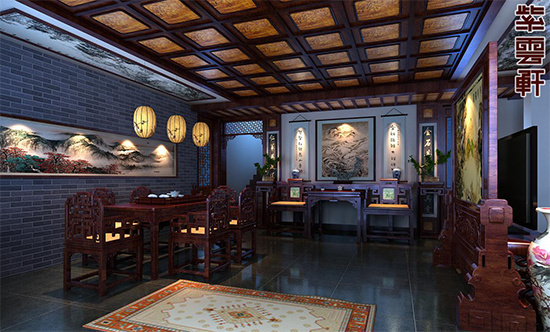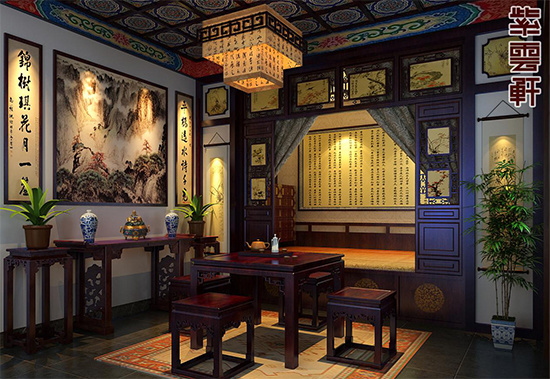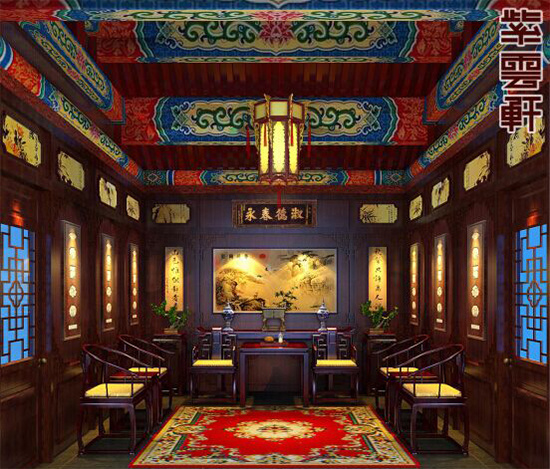|

# 中式装修的红木家具该挑选什么木材?

大家看到很多中式装修建筑，在选择家具方面很多都选择红木家具，红木家具总是以典雅美观的造型出现在我们眼前，红木家具不仅结构协调而且中式古典韵味十足，于中式设计气质相得益彰，相辅相成。紫云轩就是一家中式设计机构，善于运用木作及家具装饰中式空间，并且成立了专业的木雕厂，可以为客户定制各种木雕和家具，以下效果图内的木作及家具均来源于紫云轩。

实木家具表面一般都能看到木材真正的纹理，朴实沉稳，偶有树结的表面也体现出清新自然的材质、既天然，又无化学污染，实木家具不仅时尚而且健康，备受现在崇尚大自然的都市人的青睐。让我们看看名贵木材的综合实力的排名吧。NO.1海南黄花梨

又称降香黄檀，颜色从浅黄到紫赤。海南独特的热带季风岛屿气候为黄花梨提供了适宜的生长环境。海南黄花梨木按颜色深浅可以分为浅黄、金黄、橘黄、红褐、赤紫、深褐等若干种，通过颜色的不同也反映出木材的比重、油性、气味的不同。颜色深则比重大油性大降香气味浓，反之颜色浅则比重小油性小降香气味稍淡。同一树木的材质越接近根部颜色越深，比重和油性越大，棕眼越小木质也越致密。NO.2紫檀木

产自印度、菲律宾、广东、马来半岛、泰国。属小乔科，落叶乔木，亦有时为常绿者，树皮灰绿色，树干多弯曲，取材很小，极难得到大直径的长树，边材狭，材质致密坚硬，入水即沉，心材鲜红或橘红色，久露空气后变紫红褐色条纹，纹理纤细浮动，变化无穷，有芳香，同时也是名贵的药材，用它做成的椅子，沙发还有疗伤的功效，是我国自古以来认为最贵重的木材。

NO.3花梨/新花梨

我国自产，福建、广东、云南均有，也大量从缅甸、泰国等地进口。木色黄赤，比黄花梨木质粗，而纹直，较黄花梨稍差，无悦人香味。锯末浸水呈绿色，手伤沾湿易感染，有微毒。NO.4鸡翅木

又称杞梓木、铁刀木、丁纹木，产于缅甸、泰国、印度、越南等东南亚国家。也分新、老两种，老鸡翅木肌理致密，紫褐色深浅相间成纹，尤其是纵切而微斜的剖面，纤细浮动，予人羽毛灿烂闪耀的感觉，酷似鸡翅膀。

鸡翅木较花梨、紫檀等木产量更少，木质纹 理又独具特色，因此以其存世量少和优美艳丽的韵味为世人所珍爱。NO.5铁力木

又称铁梨木，石盐，铁棱，铁栗木。它是几种硬性木材树种中长得最高大、价值又较低廉的一种。大常绿乔木，树干直立，高可十余丈，直径达丈许，原产印度。铁力木与鸡翅木的色彩极为相似。实际上，铁力木木质纹糙粗，鬃眼显著，和鸡翅木不难分辨。NO.6乌木

又称乌文木、乌角，黑木等，产地印度、印尼、泰国、缅甸等国。心材为黑色（纯黑色或略逞绿玉色）及不规则黑色，生长年轮不明显，管空极小，木材有光泽，无特殊气味和滋味，结构细而匀，材质硬重，有油脂感，沉于水，色黑而甚脆，似紫檀而更加细密，大件的绝少。

NO.7黑檀木

产地印度尼西亚，上海人称其为绿木，特征及材性同乌木相似，纹理直至浅交错，结构细密、硬重，有油脂感，通常沉于水。

NO.8紫榆

现在最常见的一种硬木，但在清中期后被广泛使用，是当黄花梨、老鸡翅木等日见匮乏之后大量进口的。又有红木之名，广东称之为“酸枝”,而红木是江浙及北方流行的名称。产于印度、泰国、缅甸、越南、老挝等东南亚国家，系黄檀属珍贵树种之一，心材橙色、浅红褐色、红褐色、紫红色、紫褐色至黑褐色，材色不均匀，深色条纹明显，材质坚硬、耐磨、沉于水。红木也有新老之分。老红木近似紫檀，但光泽较暗，颜色较淡，质地致密也较逊，有香气，但不及黄花梨芬郁。新红木颜色赤黄，有花纹，有时颇似黄花梨，现在还大量进口。

NO.9瘿木

亦称影木，影木不是某一特定树种，而是泛指树木生病后所生的瘿瘤，为木质增生的结果。其木多节，缩蹙成山水人物鸟兽的纹案，有的木纹结成小葡萄纹及茎叶之状，名曰“满架葡萄”,极富观赏性，是最好的装饰材料，瘿木品种众多，有华木瘿、楠木瘿、榆木瘿、樟木瘿、花梨瘿等，其中又以花梨瘿最为名贵。

NO.10榉木

大叶榉树，木材坚致，色纹并美，其老龄而木材带赤色者，特名为血榉。北京人称榉木为南榆，传世南榆家具因造型纯为明式，制作手法又与黄花梨、鸡翅木等家具无殊，有的民间气息较浓，别具风格，其历史与艺术价值实不应在其他贵重木材之下。

NO.11楠木

楠木有多种，色泽淡雅匀整，伸缩性小，容易操作而耐久稳定，是非硬性木材中最好的一种。明及清前期家具除有整体用楠木者外，常与几种硬性木材配合使用。楠木还有一个特点，即除华木外，其结瘿生纹多于其他树木，因而明及清前期家具用在显著地位的瘿木，多数为楠木瘿子。

楠木有三种：一是香楠，木微紫而带清香，纹理也很美观；二是金丝楠，木纹里有金丝，是楠木中最好的一种，更为难得的是，有的楠木材料结成天然山水人物花纹。三是水楠，木质较软，多用其制作家具。

紫云轩中式装修设计机构作为中式装修装饰设计行业内一家专门从事中式设计、中式装修、中式家具、中式配饰为一体化的专业设计机构，立足北京，业务辐射全国。紫云轩并于2013年于北京成立专业的木雕厂，并和国内专业的砖雕厂，石雕厂以及中式灯饰、中式艺术壁纸企业达成长期合作，实现施工的传统工艺以及材料的一体化服务。

`声明：本文由入驻焦点开放平台的作者撰写，除焦点官方账号外，观点仅代表作者本人，不代表焦点立场错误信息举报电话： 400-099-0099，邮箱：jubao@vip.sohu.com，或点此进行意见反馈，或点此进行举报投诉。`A B C D E F G H J K L M N P Q R S T W X Y Z
A - B - C - D - E
• A
• 鞍山
• 安庆
• 安阳
• 安顺
• 安康
• 澳门
• B
• 北京
• 保定
• 包头
• 巴彦淖尔
• 本溪
• 蚌埠
• 亳州
• 滨州
• 北海
• 百色
• 巴中
• 毕节
• 保山
• 宝鸡
• 白银
• 巴州
• C
• 承德
• 沧州
• 长治
• 赤峰
• 朝阳
• 长春
• 常州
• 滁州
• 池州
• 长沙
• 常德
• 郴州
• 潮州
• 崇左
• 重庆
• 成都
• 楚雄
• 昌都
• 慈溪
• 常熟
• D
• 大同
• 大连
• 丹东
• 大庆
• 东营
• 德州
• 东莞
• 德阳
• 达州
• 大理
• 德宏
• 定西
• 儋州
• 东平
• E
• 鄂尔多斯
• 鄂州
• 恩施
F - G - H - I - J
• F
• 抚顺
• 阜新
• 阜阳
• 福州
• 抚州
• 佛山
• 防城港
• G
• 赣州
• 广州
• 桂林
• 贵港
• 广元
• 广安
• 贵阳
• 固原
• H
• 邯郸
• 衡水
• 呼和浩特
• 呼伦贝尔
• 葫芦岛
• 哈尔滨
• 黑河
• 淮安
• 杭州
• 湖州
• 合肥
• 淮南
• 淮北
• 黄山
• 菏泽
• 鹤壁
• 黄石
• 黄冈
• 衡阳
• 怀化
• 惠州
• 河源
• 贺州
• 河池
• 海口
• 红河
• 汉中
• 海东
• 怀来
• I
• J
• 晋中
• 锦州
• 吉林
• 鸡西
• 佳木斯
• 嘉兴
• 金华
• 景德镇
• 九江
• 吉安
• 济南
• 济宁
• 焦作
• 荆门
• 荆州
• 江门
• 揭阳
• 金昌
• 酒泉
• 嘉峪关
K - L - M - N - P
• K
• 开封
• 昆明
• 昆山
• L
• 廊坊
• 临汾
• 辽阳
• 连云港
• 丽水
• 六安
• 龙岩
• 莱芜
• 临沂
• 聊城
• 洛阳
• 漯河
• 娄底
• 柳州
• 来宾
• 泸州
• 乐山
• 六盘水
• 丽江
• 临沧
• 拉萨
• 林芝
• 兰州
• 陇南
• M
• 牡丹江
• 马鞍山
• 茂名
• 梅州
• 绵阳
• 眉山
• N
• 南京
• 南通
• 宁波
• 南平
• 宁德
• 南昌
• 南阳
• 南宁
• 内江
• 南充
• P
• 盘锦
• 莆田
• 平顶山
• 濮阳
• 攀枝花
• 普洱
• 平凉
Q - R - S - T - W
• Q
• 秦皇岛
• 齐齐哈尔
• 衢州
• 泉州
• 青岛
• 清远
• 钦州
• 黔南
• 曲靖
• 庆阳
• R
• 日照
• 日喀则
• S
• 石家庄
• 沈阳
• 双鸭山
• 绥化
• 上海
• 苏州
• 宿迁
• 绍兴
• 宿州
• 三明
• 上饶
• 三门峡
• 商丘
• 十堰
• 随州
• 邵阳
• 韶关
• 深圳
• 汕头
• 汕尾
• 三亚
• 三沙
• 遂宁
• 山南
• 商洛
• 石嘴山
• T
• 天津
• 唐山
• 太原
• 通辽
• 铁岭
• 泰州
• 台州
• 铜陵
• 泰安
• 铜仁
• 铜川
• 天水
• 天门
• W
• 乌海
• 乌兰察布
• 无锡
• 温州
• 芜湖
• 潍坊
• 威海
• 武汉
• 梧州
• 渭南
• 武威
• 吴忠
• 乌鲁木齐
X - Y - Z
• X
• 邢台
• 徐州
• 宣城
• 厦门
• 新乡
• 许昌
• 信阳
• 襄阳
• 孝感
• 咸宁
• 湘潭
• 湘西
• 西双版纳
• 西安
• 咸阳
• 西宁
• 仙桃
• 西昌
• Y
• 运城
• 营口
• 盐城
• 扬州
• 鹰潭
• 宜春
• 烟台
• 宜昌
• 岳阳
• 益阳
• 永州
• 阳江
• 云浮
• 玉林
• 宜宾
• 雅安
• 玉溪
• 延安
• 榆林
• 银川
• Z
• 张家口
• 镇江
• 舟山
• 漳州
• 淄博
• 枣庄
• 郑州
• 周口
• 驻马店
• 株洲
• 张家界
• 珠海
• 湛江
• 肇庆
• 中山
• 自贡
• 资阳
• 遵义
• 昭通
• 张掖
• 中卫

1室1厅1厨1卫1阳台

1
2
3
4
5

0
1
2

1

1

0
1
2
3报名成功，资料已提交审核A B C D E F G H J K L M N P Q R S T W X Y Z
A - B - C - D - E
• A
• 鞍山
• 安庆
• 安阳
• 安顺
• 安康
• 澳门
• B
• 北京
• 保定
• 包头
• 巴彦淖尔
• 本溪
• 蚌埠
• 亳州
• 滨州
• 北海
• 百色
• 巴中
• 毕节
• 保山
• 宝鸡
• 白银
• 巴州
• C
• 承德
• 沧州
• 长治
• 赤峰
• 朝阳
• 长春
• 常州
• 滁州
• 池州
• 长沙
• 常德
• 郴州
• 潮州
• 崇左
• 重庆
• 成都
• 楚雄
• 昌都
• 慈溪
• 常熟
• D
• 大同
• 大连
• 丹东
• 大庆
• 东营
• 德州
• 东莞
• 德阳
• 达州
• 大理
• 德宏
• 定西
• 儋州
• 东平
• E
• 鄂尔多斯
• 鄂州
• 恩施
F - G - H - I - J
• F
• 抚顺
• 阜新
• 阜阳
• 福州
• 抚州
• 佛山
• 防城港
• G
• 赣州
• 广州
• 桂林
• 贵港
• 广元
• 广安
• 贵阳
• 固原
• H
• 邯郸
• 衡水
• 呼和浩特
• 呼伦贝尔
• 葫芦岛
• 哈尔滨
• 黑河
• 淮安
• 杭州
• 湖州
• 合肥
• 淮南
• 淮北
• 黄山
• 菏泽
• 鹤壁
• 黄石
• 黄冈
• 衡阳
• 怀化
• 惠州
• 河源
• 贺州
• 河池
• 海口
• 红河
• 汉中
• 海东
• 怀来
• I
• J
• 晋中
• 锦州
• 吉林
• 鸡西
• 佳木斯
• 嘉兴
• 金华
• 景德镇
• 九江
• 吉安
• 济南
• 济宁
• 焦作
• 荆门
• 荆州
• 江门
• 揭阳
• 金昌
• 酒泉
• 嘉峪关
K - L - M - N - P
• K
• 开封
• 昆明
• 昆山
• L
• 廊坊
• 临汾
• 辽阳
• 连云港
• 丽水
• 六安
• 龙岩
• 莱芜
• 临沂
• 聊城
• 洛阳
• 漯河
• 娄底
• 柳州
• 来宾
• 泸州
• 乐山
• 六盘水
• 丽江
• 临沧
• 拉萨
• 林芝
• 兰州
• 陇南
• M
• 牡丹江
• 马鞍山
• 茂名
• 梅州
• 绵阳
• 眉山
• N
• 南京
• 南通
• 宁波
• 南平
• 宁德
• 南昌
• 南阳
• 南宁
• 内江
• 南充
• P
• 盘锦
• 莆田
• 平顶山
• 濮阳
• 攀枝花
• 普洱
• 平凉
Q - R - S - T - W
• Q
• 秦皇岛
• 齐齐哈尔
• 衢州
• 泉州
• 青岛
• 清远
• 钦州
• 黔南
• 曲靖
• 庆阳
• R
• 日照
• 日喀则
• S
• 石家庄
• 沈阳
• 双鸭山
• 绥化
• 上海
• 苏州
• 宿迁
• 绍兴
• 宿州
• 三明
• 上饶
• 三门峡
• 商丘
• 十堰
• 随州
• 邵阳
• 韶关
• 深圳
• 汕头
• 汕尾
• 三亚
• 三沙
• 遂宁
• 山南
• 商洛
• 石嘴山
• T
• 天津
• 唐山
• 太原
• 通辽
• 铁岭
• 泰州
• 台州
• 铜陵
• 泰安
• 铜仁
• 铜川
• 天水
• 天门
• W
• 乌海
• 乌兰察布
• 无锡
• 温州
• 芜湖
• 潍坊
• 威海
• 武汉
• 梧州
• 渭南
• 武威
• 吴忠
• 乌鲁木齐
X - Y - Z
• X
• 邢台
• 徐州
• 宣城
• 厦门
• 新乡
• 许昌
• 信阳
• 襄阳
• 孝感
• 咸宁
• 湘潭
• 湘西
• 西双版纳
• 西安
• 咸阳
• 西宁
• 仙桃
• 西昌
• Y
• 运城
• 营口
• 盐城
• 扬州
• 鹰潭
• 宜春
• 烟台
• 宜昌
• 岳阳
• 益阳
• 永州
• 阳江
• 云浮
• 玉林
• 宜宾
• 雅安
• 玉溪
• 延安
• 榆林
• 银川
• Z
• 张家口
• 镇江
• 舟山
• 漳州
• 淄博
• 枣庄
• 郑州
• 周口
• 驻马店
• 株洲
• 张家界
• 珠海
• 湛江
• 肇庆
• 中山
• 自贡
• 资阳
• 遵义
• 昭通
• 张掖
• 中卫• 手机• 分享
• 设计
免费设计
• 计算器
装修计算器
• 入驻
合作入驻
• 联系
联系我们
• 置顶
返回顶部